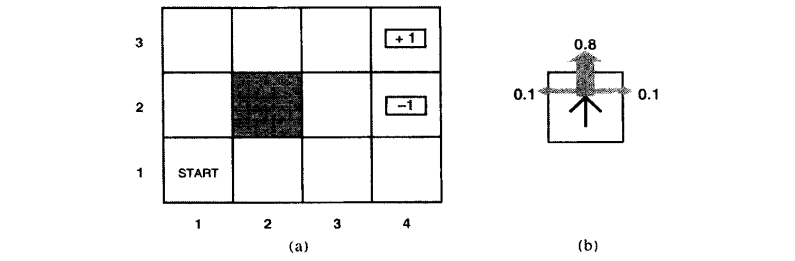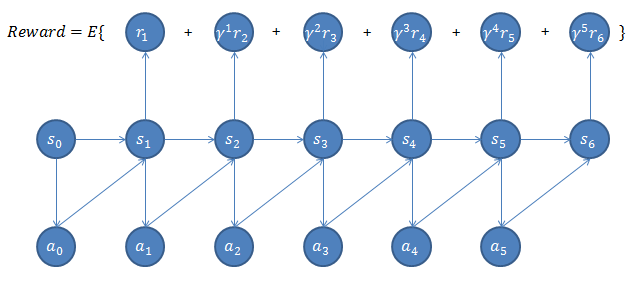## 强化学习与马尔科夫决策过程

2015年11月17日

### 一个栗子### 马尔科夫决策过程

$S$：表示状态集合，例如上例中4x3的每个环境$\{(i,j)|i=1,2,3,4,j=1,2,3\}$。自动直升机系统中的所有可能的位置、方向等。

$A$：表示一组动作集合，例如上例中的（上、下、左、右），自动直升机系统中的让飞机向前，向后等。

$P_{sa}$：状态转移概率，表示在当前$s \in S$状态下，通过执行动作$a \in A$后转移到其他状态的概率分布。例如上例中，$P_{(1,1) 上}$表示智能体在状态(1,1)执行向上的动作后转移到状态(1,2)，(2,1)的概率分布。

$\gamma \in [0,1)$：阻尼系数，表示的是随着时间的推移回报率的折扣。

$R:S \times A \mapsto \mathbb{R}$：回报函数，有时回报函数是只与$S$有关的函数，$R$重写为$R:S \mapsto \mathbb{R}$。相当于上例中对每个状态上赋予的回报值。

MDP的动态过程如下：智能体在状态$s_{0}$选择某个动作$a_{0} \in A$，智能体根据概率$P_{s_{0}a_{0}}$转移到状态$s_{1}$，然后执行动作$a_{1}$，...如此下去我们可以得到这样的过程：

$$s_{0} \stackrel{a_{0}}{\longrightarrow} s_{1} \stackrel{a_{1}}{\longrightarrow} s_{2} \stackrel{a_{2}}{\longrightarrow} s_{3} \stackrel{a_{3}}{\longrightarrow} ···$$

$$R(s_{0},a_{0})+\gamma R(s_{1},a_{1})+\gamma^{2}R(s_{2},a_{2})+···$$

$$R(s_{0})+\gamma R(s_{1})+\gamma^{2}R(s_{2})+···$$

$$Reward=E[R(s_{0})+\gamma R(s_{1})+\gamma^{2}R(s_{2})+···]$$### 进一步数学表示

$$V^{\pi}(s)=E[R(s_{0})+\gamma R(s_{1})+\gamma^{2}R(s_{2})+···|s_{0}=s,\pi]$$

$$V^{\pi}(s)=R(s)+\gamma \sum_{s' \in S}P_{s\pi(s)}(s')V^{\pi}(s')$$

$$V^{*}(s)=\max_{\pi}V^{\pi}(s)$$

$$V^{*}(s)=R(s)+\max_{a \in A}\gamma\sum_{s' \in S}P_{sa}(s')V^{*}(s')$$

$$V^{*}(s)=\max_{a}Q(s,a)$$

$$\pi^{*}(s)=arg\max_{a \in A}\sum_{s' \in S}P_{sa}(s')V^{*}(s')$$

### 值迭代方法和策略迭代方法

#### 值迭代方法

1 将每一个状态$s$的值函数$V(s)$初始化为0

2 循环直至收敛{

对于每一个状态$s$，对$V(s)$做更新

$V(s):=R(s)+\max_{a \in A}\gamma\sum_{s'}V(s')$

}

#### 策略迭代方法

1 随机初始化话一个$S$到$A$的映射$\pi$

2 循环直至收敛{

2.1 令$V:=V^{\pi}$

2.2 对每一个状态s,对$\pi(s)$做更新

$\pi(s):=arg\max_{a \in A}\sum_{s'}P_{sa}(s')V(s')$

}

2.2是根据2.1步的结果，挑选出当前状态$s$下最优的动作$a$来更新$\pi(s)$。

### MDP中的参数估计

$$s_{0}^{(1)} \stackrel{a_{0}^{(1)}}{\longrightarrow} s_{1}^{(1)} \stackrel{a_{1}^{(1)}}{\longrightarrow} s_{2}^{(1)} \stackrel{a_{2}^{(1)}}{\longrightarrow} s_{3}^{(1)} \stackrel{a_{3}^{(1)}}{\longrightarrow} ···$$

$$s_{0}^{(2)} \stackrel{a_{0}^{(2)}}{\longrightarrow} s_{1}^{(2)} \stackrel{a_{1}^{(2)}}{\longrightarrow} s_{2}^{(2)} \stackrel{a_{2}^{(2)}}{\longrightarrow} s_{3}^{(2)} \stackrel{a_{3}^{(2)}}{\longrightarrow} ···$$

$$···$$

$$P_{sa}(s')=\frac{\#times\ took\ we\ action\ a\ in\ state\ s\ and\ got\ to\ s'}{\#times\ we\ took\ action\ a\ in\ state\ s}$$

1 随机初始化话一个$S$到$A$的映射$\pi$

2 循环直至收敛{

2.1 在MDP中执行策略$\pi$一定次数

2.2 通过2.1得到的样本估计$P_{sa}$（和$R$，需要的话）

2.3 使用上一节提到的值迭代方法和估计得到的参数来更新$V$

2.4 对于得到的$V$更新得到更优的策略$\pi$

}

### 参考文献

 机器学习公开课-讲义-马尔科夫决策过程.Andrew Ng

 机器学习公开课-视频-马尔科夫决策过程.Andrew Ng

 人工智能：一种现代方法

 机器学习.Tom M.Mitchell